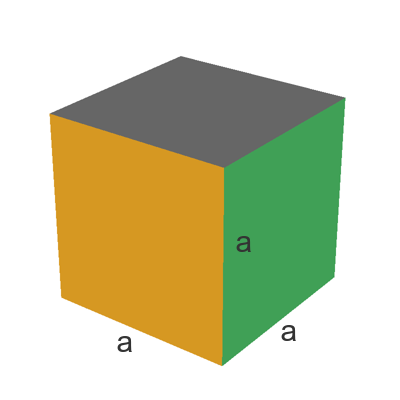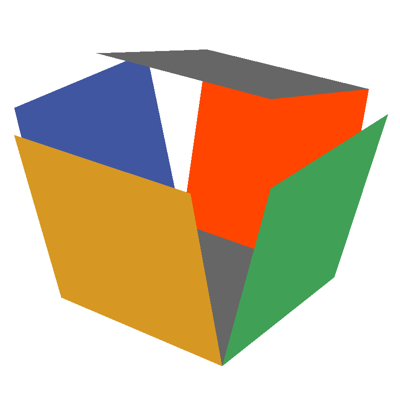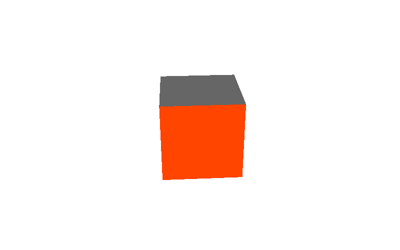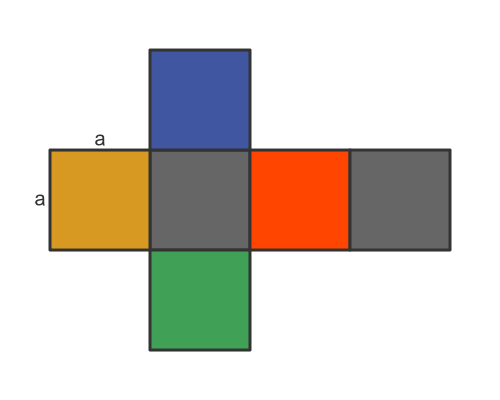# Cube surface area and net

Martin McBride
2021-02-11

Here is a cube of side $a$:## Cube net

We find the net of a cube by unfolding it, like this:This animation shows the cube being completely unfolded:## Cube surface area

The surface area of the cube is the total area of all its faces. Assuming the side of the cube has length $a$, each face has an area of $a^2$:Since there are 6 faces, the total surface area is:

$$area = 6 a^2$$